Small Squares

There are many algorithms for finding the prime factors of a large integer n, but the most effective are based on the simple idea of finding integers x and y satisfying x2y2 (mod n). Then (x + y)(xy) ≡ 0 (mod n) and there is a good chance that gcd(x + y, n) will be a non-trivial factor of n. Different factorization algorithms use different methods to find x and y that have the same square modulo n, ranging from Fermat’s method which can be worse than trial division to the number field sieve which is the best known factoring algorithm. A simple method is to randomly choose an integer x in the range √n < x < n and check whether x2 mod n is a square integer y2.

Example: This article will use as a running example the 32-bit integer n = 2,768,439,589 which is the product of the two primes p = 57,641 and q = 48,029. Suppose x is randomly chosen to be 267,857,730. Then x2 mod n = 9, and therefore x2 ≡ 32 (mod n) and a non-trivial factor is recovered by gcd(x + 3, n) = q. □

The simple method can be greatly improved by using a sieve, which relaxes the requirement that x2 mod n is a square, and instead requires it to be a product of elements of a factor base FB = {-1} ∪ { p | p is a prime and pB }. Integers satisfying this condition are called B-smooth, and after discovering |FB| + 1 B-smooth integers of the form xi2 mod n linear algebra can be used to find a subset I ⊆ {1, …, |FB| + 1} such that ΠiI (xi2 mod n) is a square integer y2. Then (ΠiI xi)2y2 (mod n) as desired.

Example: Let F11 = {-1,2,3,5,7,11} and suppose the following 11-smooth integers have been discovered:

• If x1 = 32,051,026 then x12 mod n = -20 = (-1)122305170110
• If x3 = 135,988,713 then x32 mod n = -210 = (-1)121315171110
• If x7 = 373,858,046 then x72 mod n = 42 = (-1)021315071110

Now modulo n

• (x1x3x7)2
• ≡  (x12 mod n)(x32 mod n)(x72 mod n)
• ≡  ((-1)122305170110)((-1)121315171110)((-1)021315071110)
• ≡  ((-1)224325272110)
• ≡  ((-1)122315171110)2
• ≡  (-420)2

and a non-trivial factor is again recovered by gcd(x1x3x7 – 420, n) = q. □

Since numbers with small absolute value are more likely to be B-smooth, most factorization algorithms using this approach are based on a particular method for generating integers x such that x2 mod n has small absolute value. The remainder of this article will describe a few different methods and a way to visualize the generated integers.

Visualizing Numbers

By the Chinese Remainder Theorem there is a one-to-one correspondence between numbers x mod n ∈ {0, …, n – 1} and pairs of numbers (x mod p, x mod q) ∈ {0, …, p – 1} × {0, …, q – 1}. Using this correspondence a number x mod n can be plotted as a circle inside a box of size p×q. The circle is colored blue for positive numbers (i.e., 2(x mod n) < n), and red for negative numbers (i.e., 2(x mod n) ≥ n). The size of the circle increases as the absolute value of x mod n decreases (where the absolute value of a number x mod n is itself if it is positive, and -x mod n if it is negative).

Example: Here is a plot of all numbers x mod n where the integer x satisfies -√n < x < √n. □Since the goal is to find integers x such that x2 mod n has small absolute value, the visualization is changed to communicate both pieces of information. The position of the circle plotted for the number x mod n is calculated as above, but the color and size of the circle is calculated from the sign and absolute value of x2 mod n.

Example: Here is a plot of all 1,352 numbers x mod n such that the absolute value of x2 mod n is at most 634. □The reflective symmetric pattern occurs because every square number x2 mod n is the square of four numbers with circles plotted at the following positions:

• (x mod p, x mod q)
• (x mod p, –x mod q)
• (-x mod p, x mod q)
• (-x mod p, –x mod q)

This provides a visual interpretation of the core factorization approach: given two of these circles that are not diagonally opposite each other, adding them will produce a point that is on the edge of the box, and the edges consist of all the integers that have a nontrivial common divisor with n.

The blue corners are the result of the integers {1, 2, …} having small positive squares. This makes the bottom left corner blue, and the reflective symmetry means that what happens in one corner happens in them all.

Beyond these features, it is striking how random the visualization appears: finding numbers with small squares is a number theory version of finding a needle in a haystack. The following three sections will each describe an algorithm for searching these kind of haystacks.

Square Roots

The first algorithm for finding integers x such that x2 mod n has small absolute value proceeds by checking integers around √(kn) for small k ∈ {1, 2, …}, since for i ∈ {0, 1, …}

• |(⌈√(kn)⌉ + i)2 mod n|
• =  |(√(kn) + (i + (⌈√(kn)⌉ – √(kn))))2 mod n|
• =  |(kn + 2(i + (⌈√(kn)⌉ – √(kn)))√(kn) + (i + (⌈√(kn)⌉ – √(kn)))2) mod n|
• =  |(2(i + (⌈√(kn)⌉ – √(kn)))√(kn) + (i + (⌈√(kn)⌉ – √(kn)))2) mod n|
• ≤  2(i + (⌈√(kn)⌉ – √(kn)))√(kn) + (i + (⌈√(kn)⌉ – √(kn)))2
• ≤  2(i + 1)√(kn) + (i + 1)2

and similarly

• |(⌊√(kn)⌋ – i)2 mod n|  ≤  2(i + 1)√(kn) + (i + 1)2

Minimizing the absolute value of x2 mod n is thus achieved by choosing integers x from a range of the form √(kn) – m < x < √(kn) + m.

Example: Here is a plot of all 20,000 integers x in the ranges √(kn) – m < x < √(kn) + m, where k ∈ {1, …, 20} and m = 500.The plot of each range around √(kn) looks like a battleship that is painted blue for the numbers greater than √(kn) and red for the numbers less than √(kn). Unfortunately, none of the numbers on the battleships are marked as larger “hits”, meaning that the absolute value of all of the squares is greater than 634. □

Checking integers close to √(kn) may seem like a simple idea, but the quadratic sieve does exactly this and is the second fastest factorization algorithm after the number field sieve. The quadratic sieve works so well because it defines another sieve algorithm that can search whole ranges at a time to identify all integers x such that x2 mod n are smooth numbers.

Continued Fractions

The second algorithm for finding integers x such that x2 mod n has small absolute value is based on continued fractions. For any real number r, its continued fraction approximation is a sequence of rationals ai/bi each having the property that

• |ai/bir|  ≤  1/bi2

If a/b is a continued fraction approximation to √n, then

• |a/b – √n|  ≤  1/b2
• ⇔  √n – 1/b2  <  a/b  <  √n + 1/b2
• ⇔  bn – 1/b  <  a  <  bn + 1/b
• ⇔  b2n – 2√n + 1/b2  <  a2  <  b2n + 2√n + 1/b2
• ⇔  -2√n + 1/b2  <  a2b2n  <  2√n + 1/b2
• ⇒  |a2b2n|  <  2√n + 1/b2
• ⇒  |a2 mod n|  <  2√n + 1/b2

The continued fraction factorization algorithm computes the sequence of continued fraction approximations ai/bi to √n and searches for smooth numbers in ai2 mod n.

Example: Here is a plot of the first 20,000 numerators ai in the sequence of continued fraction approximations to √n.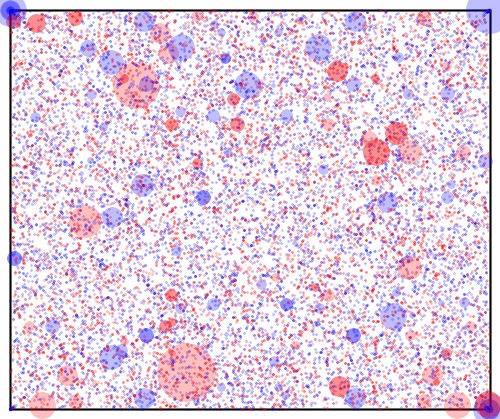The visualization clearly shows two differences between the continued fraction factorization algorithm and the quadratic sieve. Firstly, the generated numbers ai mod n are not clustered in groups, but rather are scattered throughout the whole range {0, …, n – 1}. Secondly, the effect of the tighter bound on the absolute value of ai2 mod n can be observed by the presence of larger circles (each marking an absolute value of at most 634). □

The Pell Equation

The third algorithm for finding integers x such that x2 mod n has small absolute value is based on the Pell equation. Given a positive integer n the Pell equation is a2 = nb2 + 1, and the goal is to find all integers a and b that solve the equation. If n is a square, then every solution must correspond to two integer squares that are distance 1 apart, which can only occur in the trivial solution a = ±1 and b = 0. If n is not a square, then there are infinitely many integer solutions which can all be derived from the smallest nontrivial solution computed by the Chakravala method. The Chakravala method generates a sequence of triples (ai, bi, ki) that satisfy the equation ai2 = nbi2 + ki. Each triple is used to generate the next triple according to a fixed rule which sets up a condition and then finds the minimal |ki| that satisfies the condition. The initial triple is (a0, b0, k0) = (m, 1, m2n) where m is chosen to be whichever of ⌊√n⌋ and ⌈√n⌉ minimizes |k0|. The sequence is guaranteed to converge to the smallest nontrivial integer solution of the Pell equation, which is the values of ai and bi when ki = 1.

Example: Given the Pell equation a2 = 61b2 + 1, here is the sequence computed by the Chakravala method:

• [(8,1,3), (39,5,-4), (164,21,-5), (453,58,5), (1523,195,4), (5639,722,-3), (29718,3805,-1), (469849,60158,-3), (2319527,296985,4), (9747957,1248098,5), (26924344,3447309,-5), (90520989,11590025,-4), (335159612,42912791,3), (1766319049,226153980,1)]

The smallest nontrivial integer solution a = 1,766,319,049 and b = 226,153,980 can be read from the last triple in the sequence. □

Note that each triple (ai, bi, ki) generated by the Chakravala method satisfies ai2ki (mod n), so the Chakravala method can be turned into a factorization algorithm by generating triples (ai, bi, ki) and searching for smooth numbers in ai2 mod n.

Example: Here is a plot of the first 20,000 integers ai from triples (ai, bi, ki) generated by the Chakravala method.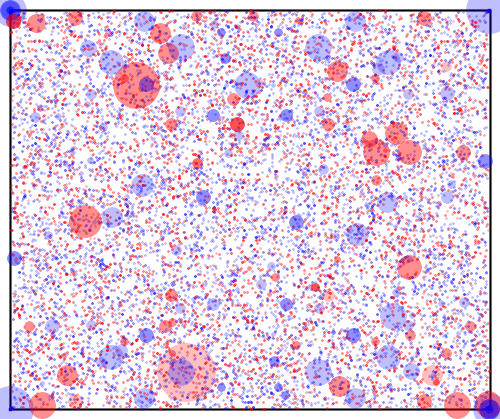The visualization is similar to the one for the numerators of the continued fraction approximations, covering the whole range {0, …, n – 1} and containing larger circles marking that the absolute value of the square is at most 634. However, closer examination shows that the Pell equation visualization is overall darker than the continued fraction visualization, indicating that the Chakravala method is finding more small squares than the continued fraction approximations. Perhaps the idea of turning the Chakravala method into a factorization algorithm is worth further study. □

King & Rook vs King

Rooks are built for survival to the endgame, which is why the basic endgame of king and rook versus a lone king is one of the first that players learn. On a standard 8×8 chessboard a king and rook can always force a checkmate against a lone king from every one of the 175,168 legal positions where it is the stronger side’s turn to move. The king and rook also win in 90% of the 223,944 legal positions where it is the lone king’s turn to move, but in 22,244 of them the lone king is either already stalemated or can immediately capture the rook. Starting from any winning position the king and rook can force checkmate in at most 16 moves with perfect play by both sides.

What about larger boards? A recently published paper defined a general strategy for n×n boards and formally proved that by following it the king and rook will always force checkmate against any defence by the lone king. The strategy in the paper works for any n greater than 3—there are no legal positions on 1×1 or 2×2 boards and the winning strategy on a 3×3 board is easy to discover. At this point we need to specify that the 50 move rule is not in force, or else the proportion of legal positions that are winning for the king and rook will become vanishingly small as the board size increases.

What about infinite boards? For a board stretching to infinity in all directions there is no hope of checkmate: from any square a lone king can move to 8 adjacent squares, and a king and rook together cannot control more than 6 of them. For the same reason a king and rook cannot checkmate a lone king on a finite board in the shape of a torus. But what about an infinite quadrant, where there is a square for every pair (x,y) of natural numbers and (1,1) is the single corner square?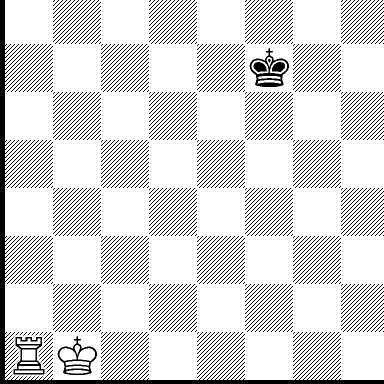A natural plan for the lone king is to run away from the corner as fast as possible, and if the rook tries to box it in then the king can move to the square diagonally adjacent to the rook and threaten to capture it, forcing it further away. The only way to stop the lone king from chasing the rook further and further away from the corner is for it to be defended by its king, and surprisingly there is a neat strategy to achieve this from any starting position. The strategy is to use the rook to box in the lone king to make it traverse two sides of a large square drawn on the board, while the other king moves along the diagonal which takes half as many moves to get to the same end point. Here are the gory details:

1. Suppose the lone king starts on the square (x,y) and the other king starts on the square (p,q).
2. The rook first makes two moves to get to the square (x + 2, n) boxing in the lone king (where n is a large number we will define later).
3. The lone king makes ny moves to get to the square (x + 1, n – 1).
4. Meanwhile, the other king makes max((n + 1) – p, (n + 1) – q) moves to get to the square (n + 1, n + 1).
5. When the lone king arrives at the square (x + 1, n – 1) to attack the rook at (x + 2, n), the rook makes one move to the square (n,n).
6. The long king makes n – (x + 2) moves to get to the square (n – 1, n – 1).

In total the king and rook together make n + 4 – min(p, q) moves, and the lone king makes 2n – (x + y + 2) moves. Thus by choosing any n ≥ 6 + x + y – min(p,q) the king and rook can arrange that when the lone king arrives at the square (n – 1, n – 1) to attack the rook at (n,n), the rook is defended by its king at (n + 1, n + 1).

Instead of immediately running toward the rook the lone king can complicate matters by trying to pin the other king to an edge and stop it moving to its rendezvous square, but the rook can always help its king to escape and once free the strategy above can be restarted. And once the lone king is boxed in by a rook defended by a king, it can be driven to the corner and checkmated with a technique similar to the one used on a standard 8×8 chessboard.

We have shown that a king and rook can force a checkmate against a lone king from any initial position on an infinite quadrant, but what about a half-infinite board that has one edge and no corners? From what starting positions can a king and rook force a checkmate against a lone king?Fox & Hounds

Fox & Hounds is a simple two-player game played on the light squares of a chessboard. Here is the initial position with the position of the fox marked by F, the position of the hounds marked by H, and the empty light squares marked by * symbols:

Fox to move
+--------+
|* * F * |
| * * * *|
|* * * * |
| * * * *|
|* * * * |
| * * * *|
|* * * * |
| H H H H|
+--------+

The fox moves first, and can move to any empty diagonally adjacent square. When it is the hounds’ turn to move one hound is chosen to move to an empty diagonally adjacent square, but hounds can only move up the board. The hounds win by blocking the fox so it can’t move:

Fox to move
+--------+
|* F H * |
| H H * *|
|* * H * |
| * * * *|
|* * * * |
| * * * *|
|* * * * |
| * * * *|
+--------+

The fox wins by escaping from the hounds, which is simplest to define as positions in which it is the hounds’ turn to move but no hound can move:

Hounds to move
+--------+
|H * H H |
| * * * *|
|* * * * |
| * * * *|
|* * * F |
| * * * H|
|* * * * |
| * * * *|
+--------+

However, in practice as soon as the fox gets around the hounds it is easy (and boring) for the fox to make waiting moves until the hounds run out of moves, so we expand our definition of winning positions for the fox to include positions where the fox has escaped into an area of two or more squares that no hound can ever reach:

Hounds to move
+--------+
|* * * * |
| * * * *|
|* * * * |
| H * H *|
|* * * * |
| F H H *|
|* * * * |
| * * * *|
+--------+

The area needs to have at least two squares so the fox can make waiting moves while the hounds exhaust all their moves. Now, before polluting your intuition by reading the following analysis, I recommend getting a feeling for the game on your own terms by playing a few rounds as the fox and as the hounds.

Solving the Game

If you’re like me there are only so many times you can play this game without needing to know who wins with perfect play, and fortunately it is feasible to compute this with the following algorithm:

1. Compute the set of reachable positions from the initial position.
2. Annotate each reachable position where the fox has won as Fox win in 0 and each reachable position where the hounds have won as Hounds win in 0.
3. Order the possible position evaluations like so:
Hounds win in 0 < Hounds win in 1 < Hounds win in 2 < ··· < Fox win in 2 < Fox win in 1 < Fox win in 0
4. For every reachable position where all the next positions have been annotated with an evaluation, if it is the fox’ turn to move then pick the maximum evaluation, and if it is the hounds’ turn to move then pick the minimum evaluation. In both cases increment the N in the win in N part of the evaluation and annotate the position with this adjusted evaluation.

This is an example of dynamic programming, and its computational complexity depends on the number of reachable positions, not the number of possible games. This is usually a huge speed-up: for example, in the classic game of noughts and crosses there are 5,478 reachable positions but 255,168 possible games.

This algorithm is coded in the solve Haskell package, and here is the result of running it on a range of possible board sizes:

 Board size Reachable positions Possible games Initial position evaluation Time Memory 2×2 1 1 Hounds win in 0 1s 200Mb 4×4 83 178 Hounds win in 8 1s 200Mb 6×6 8,175 ~1011 Fox win in 21 2s 200Mb 8×8 709,868 ~1029 Hounds win in 44 3m 650Mb 10×10 69,575,678 ~1055 Hounds win in 72 8h 41Gb

The performance results were gathered using GHC version 8.0.1 on an Intel(R) Xeon(R) Gold 6136 CPU @ 3.00GHz. The exact number of possible games for Fox & Hounds played on a standard 8×8 board is 360,552,037,329,667,882,019,232,833,884. If you’re wondering why the hounds win in zero moves on a 2×2 board, it is because the poor fox is already trapped in the initial position:

Fox to move
+--+
|F |
| H|
+--+

Designing an AI Player

Once the game was solved, my plan was to use the solution to create a strong Fox & Hounds AI player on a standard 8×8 board. And indeed, when faced with a winning position, the game solution does make it easy for an AI player to win the game. But what should an AI player do when faced with a losing position? For example, when playing as the fox from the initial position?

When faced with a hard problem, my mathematician instincts tell me to solve an easier problem instead. So let’s begin with the problem of how to play as the hounds in a losing position. To make the discussion more concrete, consider the first reachable position that is losing for the hounds, which we call the opposite position:

Fox to move
+--------+
|* * * * |
| * F * *|
|* * * * |
| * * * *|
|* * * * |
| * * * *|
|* * * H |
| H H * H|
+--------+
Evaluation: Fox win in 29

Hounds opening tip: don’t play this as your first move! Observe that this is a win in 29 moves for the fox, so with best play the hounds can delay defeat for a long time. Since the game stops as soon as the fox escapes, the N in the evaluation Fox win in N is a useful positional measure, and we use this to design a strategy for the hounds in a losing position: the AI player simply plays to delay defeat as long as possible. This same strategy is also used in solved chess endgames to create AI players that are hard to defeat when defending lost endgames such as Queen vs Rook. Try it for yourself on the opposite position, or this tricky position that is also losing for the hounds.

What Does the Fox Play?

So much for the easier problem. Unfortunately, the same strategy does not result in a strong fox AI player when playing in a losing position, but the reason why is instructive. Most games that the hounds win take about the same number of moves, because all the hounds have to move all the way up the board to trap the fox. Thus a fox playing to delay defeat will stay away from the hounds to avoid any early traps, and wait for the hounds to simply march up the board in a line for an easy win.

This last part contains the kernel of an idea for a fox strategy. First, call a position a FoxBox if the fox is contained by the hounds, such as the initial position or any of the following:

+--------+    +--------+    +--------+
|* * * * |    |* * H * |    |H * H F |
| * F * *|    | F * * *|    | * * H *|
|* * * * |    |* * H * |    |* * * H |
| * * * *|    | H H * *|    | * * * *|
|* * H H |    |* * * * |    |* * * * |
| H H * *|    | * * * *|    | * * * *|
|* * * * |    |* * * * |    |* * * * |
| * * * *|    | * * * *|    | * * * *|
+--------+    +--------+    +--------+

A simple strategy for the hounds is to play moves that maintain FoxBox positions whenever possible, and if the fox forces a non-FoxBox position then return to a FoxBox position at the earliest opportunity. Thus, when the fox is faced with a losing position, to make winning the game as complicated as possible for the hounds, the fox should avoid FoxBox positions.

The dynamic programming technique can be repeated to calculate the number of moves B required for the hounds to force a FoxBox position. Since every won position for the hounds is a FoxBox position, the B value will be finite in every position that is losing for the fox. Unfortunately, when used as a positional measure, the B value offers no guidance for the fox in FoxBox positions (such as the initial position).

A better positional measure is the maximum B value that the fox can force over the course of the whole game starting from the current position, and this can be calculated by dynamic programming yet again. When playing as the fox in a losing position, the strategy of the AI player is to maximize this quantity, which will hopefully lead to a position far from a FoxBox in which the hounds will make a mistake. For example, the B value in the initial position is 0, because it is already a FoxBox position, but the maximum value that the fox can force over the course of the game is 6, and this provides the motivation for the fox to start harassing the hounds as soon as possible.

The Fairest Fuzz Factor

At this point we have defined the strategy of the AI player when playing in a losing position as the fox or the hounds. To make the game interesting, when playing in a winning position the AI player also plays to maximize the same quantity as the losing side, but restricted to moves that preserve the win. Thus, when playing as the fox in a winning position, the AI plays toward the longest possible victory, and when playing as the hounds in a winning position, the AI plays toward the maximum finite B value.

The AI player also includes a fuzz factor to make the game more interesting: with probability p it does not follow its defined strategy but instead picks a move at random from the available moves. With the addition of a fuzz factor p > 0 a fox can now win from the initial position against an AI player, and using dynamic programming (of course) it is possible to calculate the probability of this happening as a function of p if it plays according to an optimal strategy. Using binary search on p the fairest fuzz factor for the AI player is determined to be p = 0.006935, which gives the fox exactly 50% chance of winning:

 Fuzz factor (p) Fox wins from initial position Hounds win from opposite position 0.000000 0.000 0.000 0.003906 0.326 0.150 0.005859 0.444 0.215 0.006836 0.495 0.245 0.006897 0.498 0.247 0.006927 0.499 0.248 0.006935 0.500 0.248 0.006943 0.500 0.249 0.006958 0.501 0.249 0.007080 0.507 0.253 0.007324 0.518 0.260 0.007812 0.541 0.275 0.015625 0.780 0.466 0.031250 0.943 0.696 0.062500 0.995 0.890 0.125000 1.000 0.979 0.250000 1.000 0.997 0.500000 1.000 0.998 1.000000 1.000 0.999

Just for fun, the table also includes the probability that the hounds win from the opposite position against a fox AI player with the given fuzz factor.

Conclusion

After solving the game, I was able to answer any question I had about the game with modest programming effort. The hardest part of constructing a strong fox AI player for losing positions was coming up with good questions to ask starting with the concept of the FoxBox. And perhaps there are better questions to ask that would result in stronger Fox & Hounds AI players. There is a lesson here for our Big Data future: even when all the data on a problem domain is available, creative work is still needed to solve real-world problems.

Surprising Theorems

Higher order logic is a logic of total functions, which just means that if you apply a function f : A → B to a value of its argument type A, it always produces a value of its result type B. There is no notion of definedness built into the logic, which can cause some confusion when using higher order logic formalizations of mathematical functions that are naturally partial. For example, all of the following are well-typed higher order logic terms:

• 0 - 1 : natural
• 1 mod 0 : natural
• 1 / 0 : real
• head [] : A list

Informally, we call these undefined terms because they don’t have a defined mathematical value, but formally speaking they must all have a value in higher order logic. It is tempting to ask what undefined terms evaluate to, but since we are working in a theorem prover rather than a programming language there isn’t a notion of evaluation. A better question is to ask what properties are true of undefined terms, and the answer is that you can prove many theorems about them:

• 0 * (0 - 1) = 0
• (1 mod 0) - (1 mod 0) = 0
• (0 - 1) + (1 mod 0) = (1 mod 0) + (0 - 1)
• 2 * (1 / 0) = (1 / 0) + (1 / 0)

Accidental Theorems

Can you spot the common pattern in all the above theorems involving undefined terms? They are all true of any element of the same type as the undefined term:

• ∀n : natural. 0 * n = 0
• ∀n : natural. n - n = 0
• ∀m n : natural. m + n = n + m
• ∀x : real. 2 * x = x + x
• ∀x : A. x = x

In other words, proving theorems like this about undefined terms gives us no more information than is already provided by their type. However, depending on how we have formalized the partial mathematical function we may be able to also prove accidental theorems about undefined terms that give us more information about them. For example, consider formalizing natural number subtraction with the following defining equations:

• ∀m. m - 0 = m
• ∀m n. suc m - suc n = m - n

This seems harmless enough: we cannot use these equations to prove that an undefined subtraction term such as 0 - 1 is equal to a particular natural number. However, we can instantiate the second equation to prove that two undefined subtraction terms are equal:

1 - 2 = 0 - 1

This is an accidental theorem, which we can check by replacing the undefined terms with arbitrary natural numbers:

∀m n : natural. m = n

Since this generalized theorem is clearly false, it demonstrates that the accidental theorem reveals more information about the undefined subtraction terms than is already known by their type.

Fortunately, it is usually straightforward to eliminate accidental theorems. In the case of subtraction we simply replace the second defining equation with the following:

∀m n. n ≤ m ⇒ suc m - suc n = m - n

After this change there can be no more accidental theorems about undefined subtraction terms, and we can derive no information about them other than they have natural number type.

Surprising Theorems

Suppose we have carefully formalized our partial mathematical functions to avoid any accidental theorems. Then we expect every theorem about the mod function to have a side-condition that ensures the second argument is non-zero, such as the following:

• ∀n a b. ¬(n = 0) ⇒ (a * b) mod n = ((a mod n) * (b mod n)) mod n
• ∀n a. a < n ⇒ a mod n = a

The reason for this side condition is that by design we have no information about the value of undefined mod terms, and so to prove properties of mod we have to rule out the case where the second argument is zero. This is satisfying, because the mathematical version of these theorems have the same side-condition to ensure that all terms are defined.

However, there are some surprising theorems in the formalization that do not have this side-condition, such as the following:

∀n a b. (a * n + b) mod n = b mod n

Why doesn’t this theorem need a side-condition that n is non-zero to avoid undefined mod terms? Well, if n is zero, then

a * n + b = a * 0 + b = 0 + b = b

reducing the left hand side of the surprising theorem to be exactly the same as the right hand side:

b mod 0 = b mod 0

Since this is true of every natural number, we can prove it for these undefined mod terms even though we have no information about their value.

Is This Shocking?

The existence of surprising theorems is a consequence of formalizing partial mathematical functions in higher order logic, in which there is no notion of definedness and every function is total. Even taking care to avoid accidental theorems, this fundamental logical mismatch leads to differences between mathematical theorems and their formalized versions.

In most situations this does not cause any problems, and it is merely a curiosity that certain theorems do not have the expected side-conditions. But in some circumstances, such as the need to export formalized proofs to environments that do have a notion of definedness, it may be critical to maintain a close correspondence between the mathematical theorems and their formalized versions. Happily, since the surprising feature of surprising theorems is that they are stronger than expected, we can simply choose to formalize deliberately weaker versions with logically unnecessary side-conditions, such as the following:

∀n a b. ¬(n = 0) ⇒ (a * n + b) mod n = b mod n

In this way we can maintain a close correspondence between the syntax of mathematical theorems and their formalized versions, if that be necessary.

The Slowest Software Development Methodology in the World

For some time now I’ve been practicing what can only be described as the slowest software development methodology in the world: a three step waltz of Prototyping, Verification and Export.

Prototyping: I begin quite normally by writing some code in Haskell, testing out different ways of solving some problem that I’m interested in. In this step I’m mainly focused on achieving a clean design with reasonable performance, and wherever possible I use existing Haskell packages (but restricted to those that have been developed with the same software development methodology). I also write QuickCheck tests to check various properties of the functions in the design. At this point I’m essentially done with the traditional coding phase, though usually I spend what might be perceived as an excessive amount of time polishing to make the design and properties as simple and clear as possible.

Verification: Next I use the HOL Light interactive theorem prover to develop a logical theory that (i) defines the types and functions in my Haskell design, and (ii) proves the QuickCheck properties as theorems that follow from the definitions. If the Haskell package depends on other packages that follow the same software development methodology then their contents must also be formalized in theories, and the logical definitions can build on each other in the same way as the Haskell definitions. This step is the most labour-intensive, and often some degree of creativity is required to formulate properties of recursive functions that can be proved by induction. This is where the effort spent polishing the prototype repays itself, since any unnecessary complexity in the design will inevitably thread its way through the proof of many properties.

Export: The final step is to use the opentheory tool to export the logical theory as a Haskell package. I first package up the HOL Light theory in OpenTheory format, by rendering its proof in a low-level article format and adding some meta-data such as the package name and the list of OpenTheory packages it depends on. There is also some Haskell-specific meta-data, such as the mapping from OpenTheory symbol names to Haskell names, and selecting which theorems should be exported as QuickCheck properties. Once that is complete the opentheory tool can automatically export the OpenTheory package as a Haskell package (in a form ready to be uploaded to Hackage).

Why Would You Do That?

At this point you might reasonably ask: why develop a Haskell package, throw it away, and then develop a logical theory only to export the same Haskell package again? Excellent question, and the rest of this post is devoted to answering it, but let’s begin with a depressing truth: it’s not the proof. Despite the countless hours and myriad creative insights you poured into that final Q.E.D., no one wants to see or even hear about your proof. Formal verification proofs are write-only. Sorry about that.

Specification: The proofs might be irrelevant, but the proved theorems constitute a precise specification of the Haskell package, grounded all the way down to standard mathematical objects formalized in higher order logic. I view Haskell as a computational platform for higher order logic (rather than higher order logic as a verification platform for Haskell), a perspective that emphasizes the consistent set of logical theories underlying the Haskell package that defines its semantics. A practical consequence of this is that any differences in the behaviour of the Haskell package and its higher order logic semantics (for example, due to bugs in the export code) must be fixed by changing the Haskell computational platform to conform to its higher order logic semantics.

Let’s take a look at an example specification for an extended GCD function egcd defined in the Haskell package opentheory-divides:

egcd :: Natural -> Natural -> (Natural, (Natural, Natural))

The following theorem about egcd is one of many proved in the corresponding logical theory natural-divides:

∀a b g s t. ¬(a = 0) ∧ egcd a b = (g,(s,t)) ⇒ t * b + g = s * a

This says that the three components of the egcd result (g, s and t) will satisfy a particular equation with its arguments (a and b), so long as the first argument a is non-zero. Side-conditions like this don’t fit naturally into the Haskell type system, and so the burden falls on programmers to discover them and code around them when necessary. This may be one reason why programmers prefer to write their own software components (with side-conditions tailored to the application) rather than reuse existing components (with unknown and/or inconvenient side-conditions). Using this software development methodology I shift the burden of discovering side-conditions to HOL Light, which will force me to prove that the first argument of egcd is non-zero in any code that relies on the above property of the result. If the code just uses the property that the result g of egcd is the greatest common divisor then HOL Light will understand that no side-condition proof is necessary, because another theorem in the logical theory proves that this is true for all arguments to egcd:

∀a b. fst (egcd a b) = gcd a b

Proof: It may be surprising to learn that verified Haskell packages can be useful as a building block to formalize mathematics in higher order logic. Once we have defined Haskell types and functions in the logic and proved that they satisfy their specification, we can build on this formal development to prove mathematical theorems that do not refer to any Haskell symbols. To continue the egcd example, suppose we have two natural numbers a and b (b is greater than 1) that are coprime (i.e., gcd a b = 1). Then we can reduce modulo b both sides of the first property of egcd to show the existence of a multiplicative inverse for a modulo b:

∀a b. 1 < b ∧ gcd a b = 1 ⇒ ∃s. (s * a) `mod` b = 1

This is a non-trivial higher order logic theorem which refers only to standard mathematical concepts, but its proof rests on the properties of the verified Haskell egcd function.

Maintenance: Software maintenance is a pain, and it is here that this software development methodology really shines (and who knows, maybe eventually cause the tortoise to pass the hare?). Formally verified software offers a new capability: by examining the logical theory of a Haskell package, we can see exactly which properties of dependent packages it relies on. We can use this information to automatically check whether or not a new version of a dependent package will preserve functional correctness: if so then extend the acceptable version range; and if not then report an error to the maintainer. The opentheory tool uses this technique to automatically generate maximal acceptable version ranges for dependent packages whenever it exports Haskell packages, removing another cognitive burden from the programmer. Earlier I said that formal verification proofs were write-only, but really all I meant is that humans won’t read them: as this version analysis shows machines are quite happy to read them and extract useful information.

Fun: Actually I retract the whole depressing truth: it is totally about the proof. Not for showing to people (humans still won’t read formal verification proofs) but rather for the pure fun of writing them. Those myriad creative insights you made over the course of countless hours? For me formal verification is as immersive as a video game, and my high scores come with a new artefact to play with and build upon. Free toy inside!

A Natural GCD Algorithm

The greatest common divisor (gcd) of two non-negative integers is the largest integer that divides both of them, so for example

gcd 15 6 == 3
gcd 21 10 == 1
gcd 10 0 == 10
gcd 0 0 == 0  -- by convention

Two numbers that have a gcd of 1 (like 21 and 10) are said to be coprime. Although in general it’s a hard problem to factor an integer into divisors

91 == 7 * 13
69 == 3 * 13

it’s an easy problem to compute the gcd of two integers

gcd 91 69 == 13

and if they are not coprime then a non-trivial divisor of both is revealed.

Here is a simple recursive definition that efficiently computes gcd:

gcd :: Integer -> Integer -> Integer
gcd a 0 = a
gcd a b = gcd b (a `mod` b)

How would we check that this definition always computes the gcd of two non-negative integers? If we define a divides relation

divides :: Integer -> Integer -> Bool
divides 0 b = b == 0
divides a b = b `mod` a == 0

then we can easily test that gcd returns some common divisor:

commonDivisorTest :: Integer -> Integer -> Bool
commonDivisorTest a b = let g = gcd a b in divides g a && divides g b

But how to check that gcd returns the greatest common divisor? Consider the linear combination g of a and b

g = s * a + t * b

where the coefficients s and t are arbitrary integers. Any common divisor of a and b must also be a divisor of g, and so if g is itself a common divisor of a and b then it must be the greatest common divisor. The extended gcd algorithm computes the coefficients s and t together with the gcd:

egcd :: Integer -> Integer -> (Integer,(Integer,Integer))
egcd a 0 = (a,(1,0))
egcd a b =
(g, (t, s - (a `div` b) * t))
where
(g,(s,t)) = egcd b (a `mod` b)

which allows us to test that egcd returns the greatest common divisor:

greatestCommonDivisorTest :: Integer -> Integer -> Bool
greatestCommonDivisorTest a b =
let (g,(s,t)) = egcd a b in
divides g a && divides g b && s * a + t * b == g

The coefficients s and t are useful for much more than checking the gcd result: for any coprime a and b we have

s * a + t * b == 1
(s * a) `mod` b == 1  -- assuming b > 1
(t * b) `mod` a == 1  -- assuming a > 1

which shows that s is the multiplicative inverse of a modulo b, and t is the multiplicative inverse of b modulo a.

Computing Over the Natural Numbers

The extended gcd algorithm is a classic integer algorithm, but what if we wanted a version that computed over the type of natural numbers? This would be useful in environments in which unsigned arithmetic is more natural and/or efficient than signed arithmetic (one such environment being the natural number theory of an interactive theorem prover, which is where this need arose).

The immediate problem is that one of the s and t coefficients returned by the extended gcd algorithm is usually a negative number, which is to be expected since they must satisfy

s * a + t * b == g

where g is generally less than both a and b. The solution is to modify the specification and ask for natural number coefficients s and t that satisfy

s * a == t * b + g

Fortunately for us, it turns out that such coefficients always exist except when a is zero (and b is non-zero).

Unfortunately for us, our modified specification is no longer symmetric in a and b. Given coprime a and b as input, s is the multiplicative inverse of a modulo b as before, but t is now the negation of the multiplicative inverse of b modulo a (the additive inverse of the multiplicative inverse—funny). This asymmetry breaks the recursion step of the extended gcd algorithm, because there is no longer a way to derive coefficients s and t satisfying

s * a == t * b + g

from coefficients s’ and t’ satisfying

s' * b == t' * (a `mod` b) + g

[If you try you’ll discover that a and b are the wrong way around.] This problem is solved by unwinding the recursion so that the algorithm makes two reductions before calling itself recursively, giving coefficients s’ and t’ satisfying

s' * (a `mod` b) == t' * (b `mod` (a `mod` b)) + g

Although this is expression is more complicated, it is now possible to define coefficients

s = s' + (b `div` (a `mod` b)) * t'
t = t' + (a `div` b) * s

which satisfy the desired equation

s * a == t * b + g

Here is the finished implementation:

egcd :: Natural -> Natural -> (Natural,(Natural,Natural))
egcd a 0 = (a,(1,0))
egcd a b =
if c == 0
then (b, (1, a `div` b - 1))
else (g, (u, t + (a `div` b) * u))
where
c = a `mod` b
(g,(s,t)) = egcd c (b `mod` c)
u = s + (b `div` c) * t

This satisfies a modified test confirming that it produces a greatest common divisor (for non-zero a):

greatestCommonDivisorTest :: Natural -> Natural -> Bool
greatestCommonDivisorTest a b =
let (g,(s,t)) = egcd a b in
divides g a && divides g b && s * a == t * b + g

And we can also check the upper bounds on the s and t coefficients (for non-zero a and b at least 2):

coefficientBoundTest :: Natural -> Natural -> Bool
coefficientBoundTest a b =
let (_,(s,t)) = egcd a b in
s < b && t < a

Application: The Chinese Remainder Theorem

The Chinese Remainder Theorem states that for coprime a and b, given an x and y satisfying

x < a
y < b

there is a unique n satisfying

n < a * b
n `mod` a == x
n `mod` b == y

But how to compute this n given natural number inputs a, b, x and y? We build on the natural number extended gcd algorithm, of course:

chineseRemainder :: Natural -> Natural -> Natural -> Natural -> Natural
chineseRemainder a b =
\x y -> (x * tb + y * sa) `mod` ab
where
(_,(s,t)) = egcd a b
ab = a * b
sa = s * a
tb = (a - t) * b

The λ expression in the body maximizes the precomputation on a and b, allowing efficient Chinese remaindering of different x and y inputs once the a and b inputs have been fixed. As with all computation over the natural numbers we must be careful with the subtraction operation (a - t) to ensure that the first argument is always larger than the second, but here we know from the coefficientBoundTest that t is always less than a.

References

All of the natural number computations in this blog post have been formally verified and are available as the Haskell package opentheory-divides.

Creative, Useful, Pure

Three essential attributes of work that gives me satisfaction:

• Creative: Or it should be automated. Sometimes automating non-creative work can itself be creative work.
• Useful: Or it will never see the light of day, because it doesn’t solve a problem that is causing someone pain.
• Pure: Or it will accumulate fat and inevitably collapse under its own weight.

Work with these three attributes I will seek out, I will give the time and attention to develop, I will ship the product and maintain it.

Large Knight’s Tours

I was at middle school when I first heard about the knight’s tour problem: place a knight on any square on a chess board, and move it to every square on the board without visiting any square more than once. A chess knight moves by jumping two squares forward and one square sideways, so a big part of the challenge is getting into and out of all the corners. I remember filling up pages and pages of squared notebooks with attempted solutions, although my record from that time was a tour that covered only 61 of the 64 squares.

Enter the Computer

Well, it’s one thing to look for knight’s tours by hand, but quite another to search for them with a computer. It is actually a fun programming exercise to search for knight’s tours: it is possible to use a standard backtracking algorithm, but it needs a couple of tricks to make it work. Firstly, if you have a choice of squares to jump to, then you should consider them in ascending order of accessibility (i.e., from how many unvisited squares could you jump to this square). This helps to ensure that you don’t waste last chances to visit squares. However, even with this trick, it is possible to make mistakes early on in the search that you can never recover from, and end up lost forever in a maze with 1051 turns and no exits. An ugly way to fix this is to assign a move budget—I chose 100 times the number of squares—and if you don’t find a solution within this many jumps then give up and start again from an empty board.

Armed with a fast computer, a search algorithm and a couple of tricks, I was finally able to realize a childhood dream and find a knight’s tour:Note that we always begin our tours in the bottom right corner, so that the search program only has to work out how to get into and out of 3 corners: that time spent filling squared notebooks taught me something!

Building Up

Once the initial problem is solved, I naturally start wondering whether we can find knight’s tours on larger boards. It is easy to modify the search program to change the board dimensions from an 8×8 square to an m×n rectangle, but as the board size increases the time it takes to find a knight’s tour grows rapidly:

 Board size (n×n) 8 10 12 14 16 18 20 22 24 26 28 30 Search time (ms) 1 3 7 11 26 51 102 225 401 701 1,074 1,738

Extrapolating these results to a 100×100 board, it seems that my search program would take over a thousand years to find a knight’s tour! Clearly I need a new approach to find knight’s tours for large boards.

Our 8×8 knight’s tour above looks like a little circuit, and drawn the same way a 100×100 knight’s tour would look like a huge circuit. But let’s take some inspiration from the way that huge circuits are designed in practice: not all at once, but rather by wiring together smaller circuits. Consider this special 6×6 knight’s tour:One reason this knight’s tour is special is because it has no start and end: it is a loop! There are probably clever ways to find knight’s tours that are loops, but I took a simple-minded approach. I modified my program to keep searching for knight’s tours until it found one where the end was a knight’s jump away from the start, and then I connected the ends of the tour to make a loop.

There is another reason that this knight’s tour is special, which is not so easy to see. You can put two of them together:then switch two of the jumps around to connect the tours together:and the result is a knight’s tour on a 12×6 board. To see that the result is indeed a tour, let’s visualize the two separate knight’s tours as loops using a schematic diagram: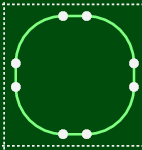And now when we perform the connection step the schematic diagram makes it clear that we have created one big loop from the two separate loops: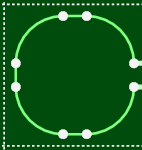The same thing also works by vertically stacking the special 6×6 knight’s tour:and connecting them like so: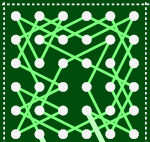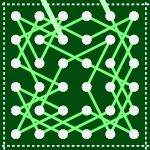Assembling Large Tours

I’ve shown how to connect special 6×6 knight’s tours together, and the next step is to connect them in an m×n grid to make a knight’s tour of a 6m×6n board. Connecting them together in a grid requires a little bit of care to avoid fragmenting the knight’s tour into disconnected paths. Here’s a schematic diagram for a 2×2 grid illustrating how tours can be fragmented into disconnected paths: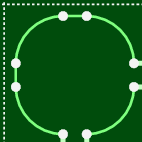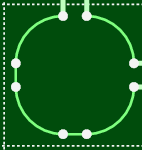One way to avoid tour fragmentation is to connect along some path through the grid that visits every 6×6 knight’s tour. I chose a spiral, starting at the bottom right of the grid, travelling anti-clockwise and ending in the middle. Here is the schematic diagram for a spiral path on a 3×3 grid: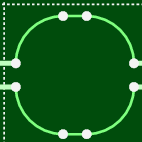And here’s the resulting knight’s tour on an 18×18 board: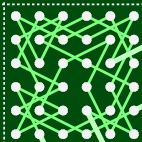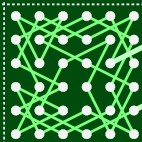Filling in the Gaps

At this stage we have the machinery to construct knight’s tours for arbitrarily large boards of dimension 6m×6n. But what about boards of other dimensions, such as the 100×100 board that we set as our target? These boards can be handled by searching for more special tours of dimensions m×n, where 6 ≤ m,n ≤ 11, and using these in place of 6×6 knight’s tours on the bottom and right edges of the grid. For example, here is a knight’s tour of a 23×23 board: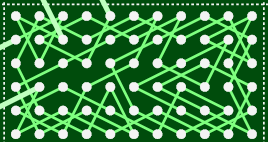This has the same 3×3 grid pattern as the 18×18 knight’s tour, but with larger pieces around the right and bottom edges. Also, the knight’s tour in the bottom right corner is not a loop—you can see one of its ends in the bottom right corner—so the schematic diagram for the assembled tour ends up looking a little different: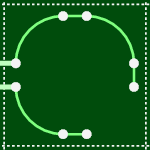In fact, it is impossible to find a knight’s tour that is a loop on this board, because it has an odd number of squares. To see why, imagine the squares of the board painted light and dark colors in a checkerboard pattern:As a knight jumps around the board it alternates between light and dark squares, and a tour of a board with an odd number of squares will contain an even number of jumps. This means the start and end squares of the tour will have the same color, and so there’s no way to jump from the end square of the tour back to the start square to close the loop.

Summary

Using these techniques you can easily assemble knight’s tours for large boards: you can view a tour of a 100×100 board or even assemble your own knight’s tour.

But these techniques only work for boards where the width and height are at least 6: there are also an infinite number of thin boards that might contain knight’s tours:Can you think of techniques for assembling knight’s tours on thin boards? ♘

Appendix

The performance results were gathered using a MacBook Air with a 1.8GHz Intel Core i7 processor and 4Gb of RAM, running OS X 10.7.5.

Explicit Laziness

This post describes a particular technique that is useful for optimizing functional programs, which I call explicit laziness. This example came about because I needed a purely functional implementation of an infinite list of primes, to demonstrate the capabilities of exporting OpenTheory logic packages to Haskell. The Sieve of Eratosthenes is a classic algorithm to compute this, and in an imperative language can be implemented with an array where it is cheap to update elements. In translating the algorithm to a purely functional language the array data structure naturally becomes a list, where it is much cheaper to update leading elements than trailing elements. This led to the idea of using explicit laziness to minimize the number of updates to trailing elements, and the result is an improved algorithm that could be backported to speed up an imperative implementation. The price of the improvement is greater complexity, but this can be hidden inside an abstract type, and the increased verification challenge can be met by the HOL Light theorem prover which is used to generate the source logic package.

Example Sieve Program

To illustrate the explicit laziness technique, we’ll use it to optimize an Haskell program for computing prime numbers based on a sieve method. This is the central Sieve data structure:

newtype Sieve =
Sieve { unSieve :: (Natural,[(Natural,Natural)]) }

Values of type Natural are non-negative integers of unbounded size. Every value Sieve (n,ps) of type Sieve must satisfy the invariant that map fst ps is the list of all prime numbers up to the perimeter n in ascending order, and the counter k in every element (p,k) of the list ps satisfies k == n `mod` p. The following examples are valid values of type Sieve:

• Sieve (9,[(2,1),(3,0),(5,4),(7,2)])
• Sieve (10,[(2,0),(3,1),(5,0),(7,3)])
• Sieve (11,[(2,1),(3,2),(5,1),(7,4),(11,0)])

The Sieve API supports two operations for creating new values of type Sieve:

initial :: Sieve
increment :: Sieve -> (Bool,Sieve)

The initial value of a Sieve is simply:

initial :: Sieve
initial = Sieve (1,[])

The increment operation takes a value Sieve (n,_) and returns a new value Sieve (n + 1, _), together with a boolean indicating whether the new perimeter n + 1 is a prime number:

increment :: Sieve -> (Bool,Sieve)
increment =
\s ->
let (n,ps) = unSieve s in
let n' = n + 1 in
let ps' = inc ps in
let b = check ps' in
let ps'' = if b then ps' ++ [(n',0)] else ps' in
(b, Sieve (n', ps''))
where
inc = map (\(p,k) -> (p, (k + 1) `mod` p))
check = all (\(_,k) -> k /= 0)

The increment operation works by adding 1 to the perimeter n <- n + 1 and every counter k <- (k + 1) `mod` p. It then checks whether any counter is zero, which happens when the perimeter is divisible by a known prime. If no counter is zero then the perimeter is a new prime, and so (n,0) is added to the end of the list.

Motivating Explicit Laziness

How can we optimize this program? Well, there are certainly many ways that we can help the compiler produce efficient target code, but before we get into that we should consider algorithmic improvements. For example, are we performing any unnecessary computation? It seems that we are, because we increment every counter even though the boolean result only needs to know whether any counter is zero. We could stop at the first zero counter and just make a note (called a closure) that the other counters need to be incremented later. This is an instance of lazy evaluation, and since Haskell is a lazy language this optimization will be performed automatically.

Unfortunately, there is still a performance problem here. Consider a time in the future when we are incrementing counters and we come to a counter with an increment closure. At this point Haskell’s lazy evaluation will execute the closure to increment the counter, and then increment it for a second time to see whether it is zero. This is functionally correct, but it would be much more efficient to add 2 to the counter rather than add 1 and then add 1 again. Even worse, it turns out that the increment closures stack up on counters during evaluation, to the point that each time we discover a new prime p we have to increment the counter k in the final list element (q,k) a total of p - q times before we can check that it is non-zero and record the new prime.

How can we avoid this inefficient behavior of adding m to a counter by adding 1 to it m times? This is a case where Haskell’s automatic laziness is not flexible enough, because whenever evaluation encounters a value with a closure, the only possible course of action is to execute the closure and then continue evaluation. There is no way to examine the closure to find out what computation it will perform. However, we can manually simulate lazy evaluation by storing data structures together with explicit notes saying what computation needs to be done before they can be used.

Demonstrating Explicit Laziness

Here is how explicit laziness works in our example. First, we extend the Sieve data structure as follows:

newtype Sieve =
Sieve { unSieve :: (Natural,[(Natural,(Natural,Natural))]) }

This is exactly the same as before, except that each list element (p,(k,j)) has an extra natural number component j, which is a note that j must be added to every counter following this one in the list.

The definition of the initial value remains the same:

initial :: Sieve
initial = Sieve (1,[])

However, the increment operation now takes into account the explicit j notes:

increment :: Sieve -> (Bool,Sieve)
increment =
\s ->
let (n,ps) = unSieve s in
let n' = n + 1 in
let (b,ps') = inc n' 1 ps in
(b, Sieve (n',ps'))
where
inc n _ [] = (True, (n,(0,0)) : [])
inc n i ((p,(k,j)) : ps) =
let k' = (k + i) `mod` p in
let j' = j + i in
if k' == 0 then (False, (p,(0,j')) : ps)
else let (b,ps') = inc n j' ps in (b, (p,(k',0)) : ps')

The inc and check functions in the previous implementation have been combined into one inc function which takes a parameter i recording how much to increment counters. Initially i is set to 1, but it absorbs the j values for the counters it visits. As soon a zero counter is discovered, the i parameter is added to the current j value and the rest of the list is untouched. This last point is crucial: explicit laziness eliminates the overhead of closures that are normally generated to update data structures not directly required for the result. For comparison with the version of the program without explicit laziness, here are the Sieve values with perimeters 9, 10 and 11:

• Sieve (9,[(2,(1,0)),(3,(0,2)),(5,(2,0)),(7,(0,0))])
• Sieve (10,[(2,(0,1)),(3,(0,2)),(5,(2,0)),(7,(0,0))])
• Sieve (11,[(2,(1,0)),(3,(2,0)),(5,(1,0)),(7,(4,0)),(11,(0,0))])

Values of the new version of the Sieve type are much harder to read than values of the original version, because the j values have to be added to every counter following it in the list. This complicates the invariant for Sieve values, but is actually a measure of the efficiency gained. For example, the j value of 2 in the Sieve value with perimeter 9 line replaces 4 counter increments in the original program: 2 for the 5 counter and another 2 for the 7 counter.

Performance Comparison

Even though explicit laziness is an algorithmic optimization, and there remain many optimizations that can be applied to both versions of the example sieve program, it is nevertheless interesting to gauge the performance effects of explicit laziness using a standard compiler. The following table presents the time taken to compute the Nth prime using both versions of the example sieve program, for various values of N:

 Nth prime 100 200 400 800 1600 3200 Without explicit laziness 0.007s 0.019s 0.068s 0.284s 1.169s 5.008s With explicit laziness 0.004s 0.008s 0.025s 0.083s 0.345s 1.495s

It is gratifying to see that the version of the example sieve program with explicit laziness is about 3x faster on this small experiment.

Summary

In this post we have described explicit laziness, a useful technique for optimizing functional programs. Like all optimization techniques its effectiveness depends on the context: automatic laziness is more flexible and guarantees that closures will be evaluated at most once, so should be the default. However, the judicious introduction of explicit laziness can eliminate closures and allow future computations to be combined into more efficient variants before executing them (a limited form of computation on computations).

Appendix

The code for the optimized version of the example sieve program is available at Hackage: this verified Haskell package is automatically generated from an OpenTheory package. The performance results were gathered using the GHC 7.4.1 compiler on a MacBook Air with a 1.8GHz Intel Core i7 processor and 4Gb of RAM, running OS X 10.7.5.